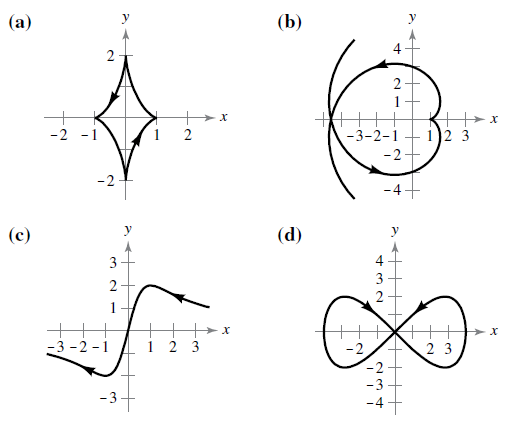Chapter 10.2, Problem 71E

Chapter
Section
Textbook Problem

# Matching In Exercises 71–74, match the set of parametric equation with its graphs. [The graphs are labeled (a), (b), (c), and (d).] Explain your reasoning.Involute of circle: x = cos θ + θ sin θ ,   y = sin θ − θ cos θTo determine
Match the curve with theequation x=cosθ+θsinθ,y=sinθθcosθ.

For figure refer to the question.

Explanation

Given: x=cosθ+θsinθ,y=sinθθcosθ

Explanation: Plot the graph on Wx Maxima by using the following steps:

Open Wx Maxima.

Click on the plot section on the toolbar.

Click on the plot 2d section under the plot toolbar

### Still sussing out bartleby?

Check out a sample textbook solution.

See a sample solution

#### The Solution to Your Study Problems

Bartleby provides explanations to thousands of textbook problems written by our experts, many with advanced degrees!

Get Started